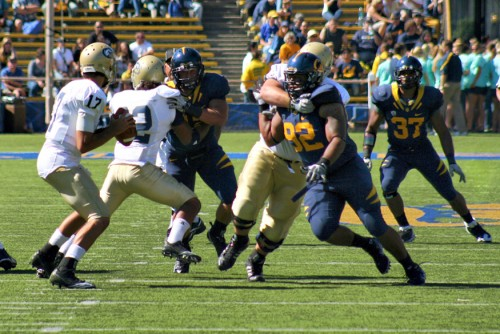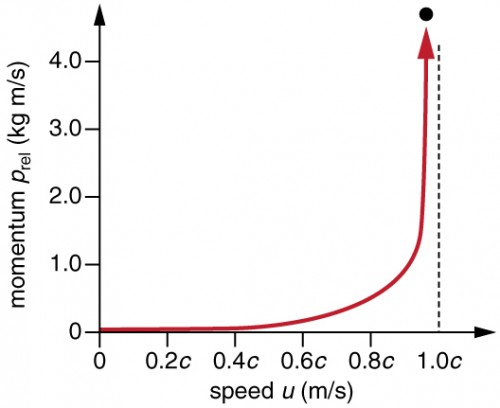## Relativistic Momentum

### Learning Objectives

By the end of this section, you will be able to:

• Calculate relativistic momentum.
• Explain why the only mass it makes sense to talk about is rest mass.Figure 1. Momentum is an important concept for these football players from the University of California at Berkeley and the University of California at Davis. Players with more mass often have a larger impact because their momentum is larger. For objects moving at relativistic speeds, the effect is even greater. (credit: John Martinez Pavliga)

In classical physics, momentum is a simple product of mass and velocity. However, we saw in the last section that when special relativity is taken into account, massive objects have a speed limit. What effect do you think mass and velocity have on the momentum of objects moving at relativistic speeds?

Momentum is one of the most important concepts in physics. The broadest form of Newton’s second law is stated in terms of momentum. Momentum is conserved whenever the net external force on a system is zero. This makes momentum conservation a fundamental tool for analyzing collisions. All of Work, Energy, and Energy Resources is devoted to momentum, and momentum has been important for many other topics as well, particularly where collisions were involved. We will see that momentum has the same importance in modern physics. Relativistic momentum is conserved, and much of what we know about subatomic structure comes from the analysis of collisions of accelerator-produced relativistic particles.

The first postulate of relativity states that the laws of physics are the same in all inertial frames. Does the law of conservation of momentum survive this requirement at high velocities? The answer is yes, provided that the momentum is defined as follows.

### Relativistic Momentum

Relativistic momentum p is classical momentum multiplied by the relativistic factor γ.

pγmu

where m is the rest mass of the object, u is its velocity relative to an observer, and the relativistic factor

$\displaystyle\gamma=\frac{1}{\sqrt{1-\frac{u^2}{c^2}}}\\$Figure 2. Relativistic momentum approaches infinity as the velocity of an object approaches the speed of light.

Note that we use u for velocity here to distinguish it from relative velocity v between observers. Only one observer is being considered here. With p defined in this way, total momentum ptot is conserved whenever the net external force is zero, just as in classical physics. Again we see that the relativistic quantity becomes virtually the same as the classical at low velocities. That is, relativistic momentum γmu becomes the classical mu at low velocities, because γ is very nearly equal to 1 at low velocities.

Relativistic momentum has the same intuitive feel as classical momentum. It is greatest for large masses moving at high velocities, but, because of the factor γ, relativistic momentum approaches infinity as u approaches c. (See Figure 2.) This is another indication that an object with mass cannot reach the speed of light. If it did, its momentum would become infinite, an unreasonable value.

### Misconception Alert: Relativistic Mass and Momentum

The relativistically correct definition of momentum as pγmu is sometimes taken to imply that mass varies with velocity: mvar = γm, particularly in older textbooks. However, note that m is the mass of the object as measured by a person at rest relative to the object. Thus, m is defined to be the rest mass, which could be measured at rest, perhaps using gravity. When a mass is moving relative to an observer, the only way that its mass can be determined is through collisions or other means in which momentum is involved. Since the mass of a moving object cannot be determined independently of momentum, the only meaningful mass is rest mass. Thus, when we use the term mass, assume it to be identical to rest mass.

Relativistic momentum is defined in such a way that the conservation of momentum will hold in all inertial frames. Whenever the net external force on a system is zero, relativistic momentum is conserved, just as is the case for classical momentum. This has been verified in numerous experiments.

In Relativistic Energy, the relationship of relativistic momentum to energy is explored. That subject will produce our first inkling that objects without mass may also have momentum.

### Check Your Understanding

What is the momentum of an electron traveling at a speed 0.985c? The rest mass of the electron is 9.11 × 10−31 kg.

#### Solution

$\displaystyle{p}=\gamma{mu}=\frac{mu}{\sqrt{1-\frac{u^2}{c^2}}}=\frac{\left(9.11\times10^{-31}\text{ kg}\right)\left(0.985\right)\left(3.00\times10^{8}\text{ m/s}\right)}{\sqrt{1-\frac{\left(0.985c\right)^2}{c^2}}}\\$ = 1.56 × 10−21 kg · m/s

## Section Summary

• The law of conservation of momentum is valid whenever the net external force is zero and for relativistic momentum. Relativistic momentum p is classical momentum multiplied by the relativistic factor γ.
• = γmu, where m is the rest mass of the object, u is its velocity relative to an observer, and the relativistic factor $\displaystyle\gamma=\frac{1}{\sqrt{1-\frac{{u}^{2}}{{c}^{2}}}}\\$.
• At low velocities, relativistic momentum is equivalent to classical momentum.
• Relativistic momentum approaches infinity as u approaches c. This implies that an object with mass cannot reach the speed of light.
• Relativistic momentum is conserved, just as classical momentum is conserved.

### Conceptual Questions

1. How does modern relativity modify the law of conservation of momentum?
2. Is it possible for an external force to be acting on a system and relativistic momentum to be conserved? Explain.

### Problems & Exercises

1. Find the momentum of a helium nucleus having a mass of 6.68 × 10−27 kg that is moving at 0.200c.
2. What is the momentum of an electron traveling at 0.980c?
3. (a) Find the momentum of a 1.00 × 109 kg asteroid heading towards the Earth at 30.0 km/s. (b) Find the ratio of this momentum to the classical momentum. (Hint: Use the approximation that $\gamma=1+\left(\frac{1}{2}\right)\left(\frac{{v}^{2}}{{c}^{2}}\right)\\$ at low velocities.)
4. (a) What is the momentum of a 2000 kg satellite orbiting at 4.00 km/s? (b) Find the ratio of this momentum to the classical momentum. (Hint: Use the approximation that $\gamma=1+\left(\frac{1}{2}\right)\left(\frac{{v}^{2}}{{c}^{2}}\right)\\$ at low velocities.)
5. What is the velocity of an electron that has a momentum of 3.04 × 10−21 kg · m/s? Note that you must calculate the velocity to at least four digits to see the difference from c.
6. Find the velocity of a proton that has a momentum of 4.48 ×−10−19 kg · m/s.
7. (a) Calculate the speed of a 1.00-μg particle of dust that has the same momentum as a proton moving at 0.999c. (b) What does the small speed tell us about the mass of a proton compared to even a tiny amount of macroscopic matter?
8. (a) Calculate γ for a proton that has a momentum of 1.00 kg · m/s. (b) What is its speed? Such protons form a rare component of cosmic radiation with uncertain origins.

## Glossary

relativistic momentum: p, the momentum of an object moving at relativistic velocity; = γmu, where m is the rest mass of the object, u is its velocity relative to an observer, and the relativistic factor

$\displaystyle\gamma=\frac{1}{\sqrt{1-\frac{{u}^{2}}{{c}^{2}}}}\\$

rest mass: the mass of an object as measured by a person at rest relative to the object

### Selected Solutions to Problems & Exercises

1. 4.09 × 10−19 kg · m/s

3. (a) 3.000000015 × 1013 kg · m/s; (b) Ratio of relativistic to classical momenta equals 1.000000005 (extra digits to show small effects)

5. 2.9957 × 108 m/s

7. (a) 1.121 × 10−8 m/s; (b) The small speed tells us that the mass of a proton is substantially smaller than that of even a tiny amount of macroscopic matter!